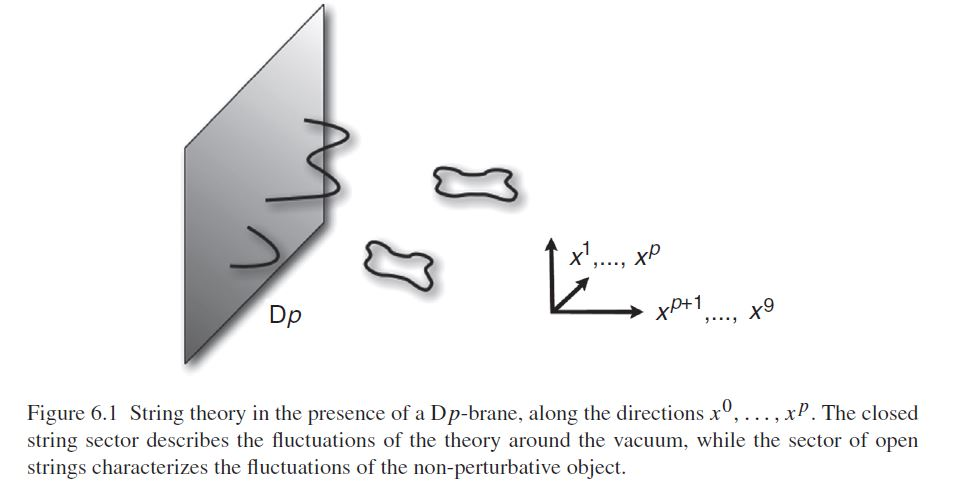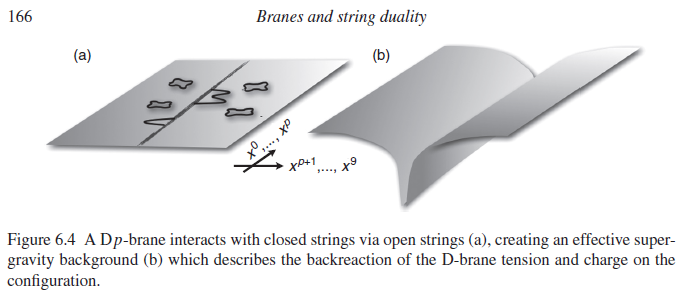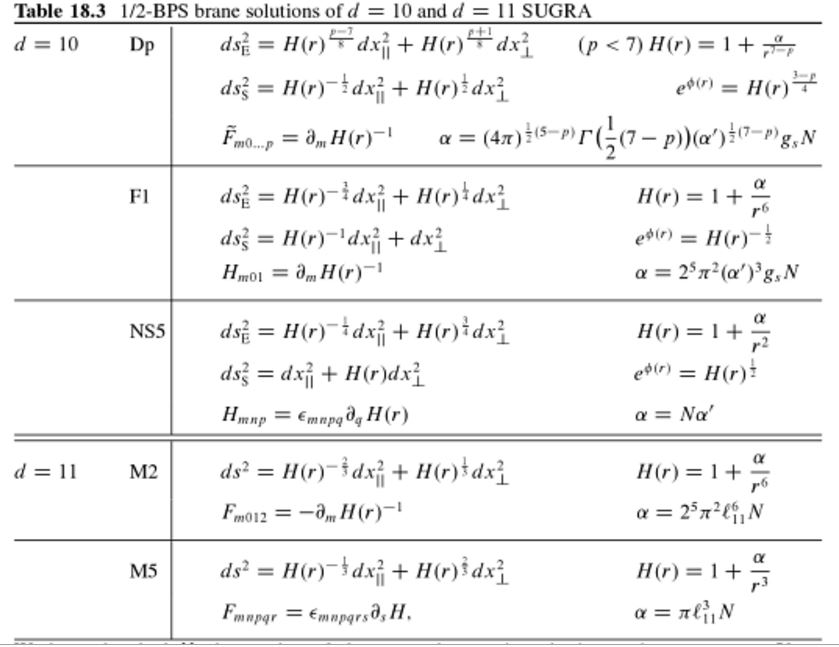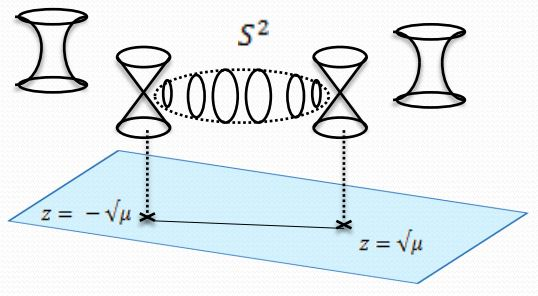Contents

# Contents

## Idea

An abstractly defined $n$-dimensional quantum field theory is a consistent assignment of state-space and correlators to $n$-dimensional cobordisms with certain structure (topological structure, conformal structure, Riemannian structure, etc. see FQFT/AQFT). In an open-closed QFT the cobordisms are allowed to have boundaries.

In this abstract formulation of QFT a D-brane is a type of data assigned by the QFT to boundaries of cobordisms.

For a broader perspective see at brane.

### In $2d$ rational CFT

A well understood class of examples is this one: among all 2-dimensional conformal field theory that case of full rational 2d CFT has been understood completely, using FFRS-formalism. It is then a theorem that full 2-rational CFTs are classified by

1. a modular tensor category $\mathcal{C}$ (to be thought of as being the category of representations of the vertex operator algebra of the 2d CFT);

2. a special symmetric Frobenius algebra object $A$ internal to $\mathcal{C}$.

In this formulation a type of brane of the theory is precisely an $A$-module in $\mathcal{C}$ (an $A$-bimodule is a bi-brane or defect line ):

the 2d cobordisms with boundary on which the theory defined by $A \in \mathcal{C}$ carry as extra structure on their connected boundary pieces a label given by an equivalence class of an $A$-module in $\mathcal{C}$. The assignment of the CFT to such a cobordism with boundary is obtained by

• triangulating the cobordism,

• labeling all internal edges by $A$

• labelling all boundary pieces by the $A$-module

• all vertices where three internal edges meet by the multiplication operation

• and all points where an internal edge hits a boundary by the corresponding action morphism

• and finally evaluating the resulting string diagram in $\mathcal{C}$.

So in this abstract algebraic formulation of QFT on the worldvolume, a brane is just the datum assigned by the QFT to the boundary of a cobordism. But abstractly defined QFTs may arise from quantization of sigma models. This gives these boundary data a geometric interpretation in some space. This we discuss in the next section.graphics grabbed from Ibanez-Uranga 12

### In $2d$ TFT

Another case where the branes of a QFT are under good mathematical control is TCFT: the (infinity,1)-category-version of a 2d TQFT.

Particularly the A-model and the B-model are well understood.

There is also a mathematical structure called string topology with D-branes. At present this is more “string inspired” than actually derived from string theory, though.

### In terms of geometric data of the $\sigma$-model background

An abstractly defined QFT (as a consistent assignment of state spaces and propagators to cobordisms as in FQFT) may be obtained by quantization from geometric data :

Such a sigma-model QFT is the quantization of an action functional on a space of maps $\Sigma \to X$ from a cobordism (“worldvolume”) $\Sigma$ to some target space $X$ that may carry further geometric data such as a Riemannian metric, or other background gauge fields.

One may therefore try to match the geometric data on $X$ that encodes the $\sigma$-model with the algebraic data of the FQFT that results after quantization. This gives a geometric interpretation to many of the otherwise purely abstract algebraic properties of the worldvolume QFT.

It turns out that if one checks which geometric data corresponds to the $A$-modules in the above discussion, one finds that these tend to come from structures that look at least roughly like submanifolds of the target space $X$. And typically these submanifolds themselves carry their own background gauge field data.

A well-understood case is the Wess-Zumino-Witten model: for this the target space $X$ is a simple Lie group $X = G$ and the background field is a circle 2-bundle with connection (a bundle gerbe) on $G$, representing the background field that is known as the Kalb-Ramond field.

In this case it turns out that branes for the sigma model on $X$ are given in the simplest case by conjugacy classes $D \subset G$ inside the group, and that these carry twisted vector bundle with the twist given by the Kalb-Ramond background bundle. These vector bundles are known in the string theory literature as Chan-Paton vector bundles . The geometric intuition is that a QFT with certain boundary condition comes from a quantization of spaces of maps $\Sigma \to G$ that are restricted to take the boundary of $\Sigma$ to these submanifolds.

More generally, one finds that the geometric data that corresponds to the branes in the algebraically defined 2d QFT is given by cocycles in the twisted differential K-theory of $G$. These may be quite far from having a direct interpretation as submanifolds of $G$.

The case of rational 2d CFT considered so far is only the best understood of a long sequence of other examples. Here the collection of all D-branes – identified with the collection of all internal modules over an internal frobenius algebra, forms an ordinary category.

More generally, at least for 2-dimensional TQFTs analogous considerations yield not just categories but stable (∞,1)-categories of boundary condition objects. For instance, for what is called the B-model 2-d TQFT the category of D-branes is the derived category of coherent sheaves on some Calabi-Yau space.

Starting with Kontsevich’s homological algebra reformulation of mirror symmetry the study of (derived) D-brane categories has become a field in its own right in pure mathematics.

… lots of further things to say …

### As black branes

In perturbative string theory, hence for small string coupling constant the D-branes are incarnated as boundary conditions for the string’s worldsheet 2d CFT, exhibiting submanifolds in spacetime. As the string coupling constant increases and becomes non-perturbative, this picture of perturbative string theory breaks down, but at low energy (large scales) now supergravity becomes a good description, and now the D-branes are incarnated as black branes.graphics grabbed from Ibanez-Uranga 12

This transition is also the key to understanding black holes in string theory.(table taken from Blumenhagen-Lüst-Theisen 13, Chapter 18.5)

## Examples

### In the WZW model

For D-branes in the WZW-model see WZW-model – D-branes.

## Properties

### As F-branes originating from M-branes

from M-branes to F-branes: superstrings, D-branes and NS5-branes

M-theory on $S^1_A \times S^1_B$-elliptic fibrationKK-compactification on $S^1_A$type IIA string theoryT-dual KK-compactification on $S^1_B$type IIB string theorygeometrize the axio-dilatonF-theory on elliptically fibered-K3 fibrationduality between F-theory and heterotic string theoryheterotic string theory on elliptic fibration
M2-brane wrapping $S_A^1$double dimensional reduction $\mapsto$type IIA superstring$\mapsto$type IIB superstring$\mapsto$$\mapsto$heterotic superstring
M2-brane wrapping $S_B^1$$\mapsto$D2-brane$\mapsto$D1-brane$\mapsto$
M2-brane wrapping $p$ times around $S_A^1$ and $q$ times around $S_B^1$$\mapsto$$p$ strings and $q$ D2-branes$\mapsto$(p,q)-string$\mapsto$
M5-brane wrapping $S_A^1$double dimensional reduction $\mapsto$D4-brane$\mapsto$D5-brane$\mapsto$
M5-brane wrapping $S_B^1$$\mapsto$NS5-brane$\mapsto$NS5-brane$\mapsto$$\mapsto$NS5-brane
M5-brane wrapping $p$ times around $S_A^1$ and $q$ times around $S_B^1$$\mapsto$$p$ D4-brane and $q$ NS5-branes$\mapsto$(p,q)5-brane$\mapsto$
M5-brane wrapping $S_A^1 \times S_B^1$$\mapsto$$\mapsto$D3-brane$\mapsto$
KK-monopole/A-type ADE singularity (degeneration locus of $S^1_A$-circle fibration, Sen limit of $S^1_A \times S^1_B$ elliptic fibration)$\mapsto$D6-brane$\mapsto$D7-branes$\mapsto$A-type nodal curve cycle degeneration locus of elliptic fibration(Sen 97, section 2)SU-gauge enhancement
KK-monopole orientifold/D-type ADE singularity$\mapsto$D6-brane with O6-planes$\mapsto$D7-branes with O7-planes$\mapsto$D-type nodal curve cycle degeneration locus of elliptic fibration(Sen 97, section 3)SO-gauge enhancement
exceptional ADE-singularity$\mapsto$$\mapsto$$\mapsto$exceptional ADE-singularity of elliptic fibration$\mapsto$E6-, E7-, E8-gauge enhancement

(e.g. Johnson 97, Blumenhagen 10)

### Characterization in terms of Dirac structures

D-branes may be identified with Dirac structures on a Courant Lie 2-algebroid over spacetime related to the type II geometry (Asakawa-Sasa-Watamura). See at Dirac structure for more on this.

### D-brane charge

In analogy to how in electromagnetism magnetic charge is given by a class in ordinary cohomology, so D-brane charge is given in (twisted) K-theory, or, if preferred, in its image under the Chern character.

The Chan-Paton bundle carried by a D-brane defines a class in twisted K-theory on the D-brane worldvolume and the D-brane charge is the push-forward (Umkehr map) of this class to spacetime, using a K-orientation of the embedding of the D-brane (a spin^c structure).

#### General

More in detail this means the following (BMRS2).

Let $X$ be a manifold regarded as spacetime and $i \colon Q \hookrightarrow X$ a submanifold regarded as the worldvolume of a D-brane. For $\nabla_B \colon X \to \mathbf{B}^2 U(1)_{conn}$ the circle 2-bundle with connection which models the background B-field, write $\chi_B \colon X \to \mathbf{B}^2 U(1)$ for the underlying circle 2-group-principal 2-bundle.

The corresponding Chan-Paton bundle (a twisted line bundle for the case of a single D-brane) is the trivialization $\xi$ in

$\array{ && Q \\ & \swarrow && \searrow^{\mathrlap{i}} \\ \ast && \swArrow_{\xi} && X \\ & \searrow && \swarrow_{\mathrlap{\chi_B}} \\ && \mathbf{B}^2 U(1) } \;\;\;\;\; \simeq \;\;\;\;\; \array{ && Q \\ & \swarrow &\downarrow& \searrow^{\mathrlap{i}} \\ \ast &\swArrow_{\xi}& \downarrow^{\mathrlap{i^\ast \chi_B}} &\swArrow_{id}& X \\ & \searrow &\downarrow& \swarrow_{\mathrlap{\chi_B}} \\ && \mathbf{B}^2 U(1) } \,.$

Assuming that $i \colon Q \to X$ is K-oriented in that for instance $X$ has a spin-structure and $Q$ a spin^c-structure, then under the groupoid convolution algebra functor $C^\ast$ this is incarnated as a Hilbert bimodule which defines a class in twisted operator K-theory, realized as the following comoposite in KK-theory

$\mathbb{C} \stackrel{\Gamma(\xi)}{\to} C(Q)_{i^\ast \chi_B} \stackrel{i_!}{\to} C(X)_{\chi_B} \,,$

where

• $C(Q)$ and $C(X)$ are the C*-algebras of functions (vanishing at infinity) on the D-brane and on spacetime, respectively;

• $C(X)_{\chi_B}$ is the groupoid convolution algebra of sections of $\chi_B$ regarded as a centrally extended groupoid over a Cech groupoid resolution of $X$ which supports a Cech cocycle for $\chi_B$, and similarly for $C(Q)_{i^\ast \chi B}$ and the pullback/restriction $i^\ast \chi_B$ of the background B-field to the brane;

• $i!$ is the push-forward (Umkehr map) dual to $i^\ast \colon C(X)_{\chi_B} \to C(Q)_{i^\ast \chi_B}$, realizes as a KK-theory class

$D_Q = i! = \in KK(C(Q), C(X)_{\chi_B}) \,.$

The corresponding D-brane charge in KK-theory is the resulting composite (relative index)

$i_!(\xi) = D_Q(\xi) \in KK(\mathbb{C}, C(X)_{\chi_B}) \simeq K^{[\chi_b]}(X)$

in twisted K-theory. Traditionally only the image of this under the Chern character

$ch \colon KK \to HL$

in real cohomology/cyclic cohomology? is considered, $ch(D_Q(\xi))$. Moreover, traditonally one thinks of first applying $ch$ to $\xi$ and then pushing forward in $HL$. By the C*-algebraic Grothendieck-Riemann-Roch theorem this gives the isomorphic expression

$ch(D_Q(\xi)) \otimes_{C(X)_{\chi_B}} \sqrt{Todd} \in HL \,,$

where on the right we have the relative Todd class. This is the form the D-brane charge was originally found in the physics literature and in which it is still often given.

(In (BMRS2, Section 4) this is discussed for the untwisted case.)

For more general discussion see at Freed-Witten anomaly – Details as well as at Poincaré duality algebra – Properties – K-Orientation and Umkehr maps.

#### Via the Atiyah-Hirzebruch spectral sequence

The Atiyah-Hirzeburch spectral sequence expresses, starting from its $E_2$ pages, K-theory classes on spacetime $X$ as kernels of certain differential acting on ordinary cohomology in all even degrees (for type IIA strings) or all odd degrees (for type IIB strings)

$E_2^{p,q} = H^p(X, KU^q(\ast)) \Rightarrow KU^\bullet(X) \,.$

Discussion of D-brane charge this way is in (Maldacena-Moore-Seiberg 01, Evslin-Sati 06).

Table of branes appearing in supergravity/string theory (for classification see at brane scan).

branein supergravitycharged under gauge fieldhas worldvolume theory
black branesupergravityhigher gauge fieldSCFT
D-branetype IIRR-fieldsuper Yang-Mills theory
$(D = 2n)$type IIA$\,$$\,$
D(-2)-brane$\,$$\,$
D0-brane$\,$$\,$BFSS matrix model
D2-brane$\,$$\,$$\,$
D4-brane$\,$$\,$D=5 super Yang-Mills theory with Khovanov homology observables
D6-brane$\,$$\,$D=7 super Yang-Mills theory
D8-brane$\,$$\,$
$(D = 2n+1)$type IIB$\,$$\,$
D(-1)-brane$\,$$\,$$\,$
D1-brane$\,$$\,$2d CFT with BH entropy
D3-brane$\,$$\,$N=4 D=4 super Yang-Mills theory
D5-brane$\,$$\,$$\,$
D7-brane$\,$$\,$$\,$
D9-brane$\,$$\,$$\,$
(p,q)-string$\,$$\,$$\,$
(D25-brane)(bosonic string theory)
NS-branetype I, II, heteroticcircle n-connection$\,$
string$\,$B2-field2d SCFT
NS5-brane$\,$B6-fieldlittle string theory
D-brane for topological string$\,$
A-brane$\,$
B-brane$\,$
M-brane11D SuGra/M-theorycircle n-connection$\,$
M2-brane$\,$C3-fieldABJM theory, BLG model
M5-brane$\,$C6-field6d (2,0)-superconformal QFT
M9-brane/O9-planeheterotic string theory
M-wave
topological M2-branetopological M-theoryC3-field on G2-manifold
topological M5-brane$\,$C6-field on G2-manifold
S-brane
SM2-brane,
membrane instanton
M5-brane instanton
D3-brane instanton
solitons on M5-brane6d (2,0)-superconformal QFT
self-dual stringself-dual B-field
3-brane in 6d

∞-Chern-Simons theory from binary and non-degenerate invariant polynomial

$n \in \mathbb{N}$symplectic Lie n-algebroidLie integrated smooth ∞-groupoid = moduli ∞-stack of fields of $(n+1)$-d sigma-modelhigher symplectic geometry$(n+1)$d sigma-modeldg-Lagrangian submanifold/ real polarization leaf= brane(n+1)-module of quantum states in codimension $(n+1)$discussed in:
0symplectic manifoldsymplectic manifoldsymplectic geometryLagrangian submanifoldordinary space of states (in geometric quantization)geometric quantization
1Poisson Lie algebroidsymplectic groupoid2-plectic geometryPoisson sigma-modelcoisotropic submanifold (of underlying Poisson manifold)brane of Poisson sigma-model2-module = category of modules over strict deformation quantiized algebra of observablesextended geometric quantization of 2d Chern-Simons theory
2Courant Lie 2-algebroidsymplectic 2-groupoid3-plectic geometryCourant sigma-modelDirac structureD-brane in type II geometry
$n$symplectic Lie n-algebroidsymplectic n-groupoid(n+1)-plectic geometry$d = n+1$ AKSZ sigma-model

(adapted from Ševera 00)

### General

The original article is

although it appears that we have modified the type II theory by adding something new to it, we are now arguing that these objects are actually intrinsic to any nonperturbative formulation of the type II theory; presumably one should think of them as an alternate representation of the black $p$-branes

General review:

A classical text describing how the physics way to think of D-branes leads to seeing that they are objects in derived categories is

Discussion with an eye towards string phenomenology is in

This can to a large extent be read as a dictionary from homological algebra terminology to that of D-brane physics.

More recent similar material with the emphasis on the K-theory aspects is

Comments on the role of D-branes in mathematical physics and mathematics is in

### On orbifolds

Review includes

• Frederik Roose, Strings and D-branes on orbifolds: from boundary states to geometry, 2001 (pdf)

• Nikolas Prezas, Aspects of branes and orbifolds in string theory, 2002 (pdf, web)

### As higher super-GS-WZW type $\sigma$-models

The Green-Schwarz sigma model with DBI action for D-branes is discussed in

Discussion of Green-Schwarz action functionals for super D-branes and the interpretation of the WZW cocycles for the D-branes as cocycles on “extended super-Minkowski spacetime” is due to

A corresponding discussion as ∞-Wess-Zumino-Witten theory and refinement of the brane scan to a “brane bouquet” of super L-∞ algebra extensions (hence in infinity-Lie theory via ∞-Wess-Zumino-Witten theory) is discussed in

### D-brane charge quantization in topological K-theory

On the conjectural D-brane charge quantization in topological K-theory:

#### Origin and basics

The idea that D-branes have Dirac charge quantization in topological K-theory originates with:

and with emphasis on the full picture of twisted differential K-theory in:

Here:

Expression of these D-brane K-theory classes via the Atiyah-Hirzebruch spectral sequence:

Specifically for D-branes in WZW models see

• Peter Bouwknegt, A note on equality of algebraic and geometric D-brane charges in WZW models (pdf)

Discussion of D-brane matrix models taking these K-theoretic effects into account (K-matrix model) is in

• T. Asakawa, S. Sugimoto, S. Terashima, D-branes, Matrix Theory and K-homology, JHEP 0203 (2002) 034 (arXiv:hep-th/0108085)

### Twisted, equivariant and differential refinement

Discussion of charge quantization in twisted K-theory for the case of non-vanishing B-field:

An elaborate proposal for the correct flavour of equivariant KR-theory needed for orientifolds is sketched in:

Discussion of full-blown twisted differential K-theory and its relation to D-brane charge in type II string theory

Discussion of full-blown twisted differential orthogonal K-theory and its relation to D-brane charge in type I string theory (on orientifolds):

#### Reviews

Discussion of D-branes in KK-theory is reviewed in

based on

In particular (BMRS2) discusses the definition and construction of D-brane charge as a generalized index in KK-theory. The discussion there focuses on the untwisted case. Comments on the generalization of this to topologicall non-trivial B-field and hence twisted K-theory is in

#### Conceptual problems

But there remain conceptual issues with the proposal that D-brane charge is in K-theory, as highlighted in

In particular, actual checks of the proposal that D-brane charge is given by K-theory, via concrete computation in boundary conformal field theory, have revealed some subtleties:

• Stefan Fredenhagen, Thomas Quella, Generalised permutation branes, JHEP 0511:004, 2005 (arXiv:hep-th/0509153)

It might surprise that despite all the progress that has been made in understanding branes on group manifolds, there are usually not enough D-branes known to explain the whole charge group predicted by (twisted) K-theory.

The closest available towards an actual check of the argument for K-theory via open superstring tachyon condensation (Witten 98, Section 3) seems to be

• Theodore Erler, Analytic Solution for Tachyon Condensation in Berkovits’ Open Superstring Field Theory, JHEP 1311 (2013) 007 (arXiv:1308.4400)

which, however, concludes (on p. 32) with:

It would also be interesting to see if these developments can shed light on the long-speculated relation between string field theory and the K-theoretic description of D-brane charge $[$75, 76, 77$]$. We leave these questions for future work.

which still lists (on p. 112) among open problems of string field theory:

“Are there topological invariants of the open string star algebra representing D-brane charges?”

#### For orbifolds in equivariant K-theory

The proposal that D-brane charge on orbifolds is measured in equivariant K-theory (orbifold K-theory) goes back to

It was pointed out that only a subgroup of equivariant K-theory can be physically relevant in

Further discussion of equivariant K-theory for D-branes on orbifolds includes the following:

Discussion of real K-theory for D-branes on orientifolds includes the following:

The original observation that D-brane charge for orientifolds should be in KR-theory is due to

and was then re-amplified in

With further developments in

Discussion of orbi-orienti-folds using equivariant KO-theory is in

Discussion of the alleged K-theory classification of D-brane charge in relation to the M-theory C-field is in

More complete discussion of double dimensional reduction of the supergravity C-field in 11d to the expected B-field and RR-field flux forms in 10d:

### Entropy

The entropy of D-branes scales with the square of their number:

Around equation (3) in

Around (3) in:

Around equation (5.5) in:

• Most of the dramatic progress in string theory over the past decade has in some way involved the degrees of freedom of D-branes, solitonic objects in string theory whose low energy physics is described by quantum field theories living on the branes. Essential to many of the applications of D-brane physics is the fact that the number of degrees of freedom of a collection of branes scales not as the number of branes, but as the square of the number of branes.

Around (1.1) in:

• Qianqian Du, Michael Strickland, Ubaid Tantary, $\mathcal{N} = 4$ supersymmetric Yang-Mills thermodynamics to order $\lambda^2$ (arXiv:2105.02101)

### For rational CFT

For exhaustive details on D-branes in 2-dimensional rational CFT see the references given at

### For topological strings

A discussion of topological D-branes in the context of higher category theory is in

### Open string worldsheet Anomaly cancellation

The need for twisted spin^c structures as quantum anomaly-cancellaton condition on the worldvolume of D-branes was first discussed in

More details are in

A clean review is provided in

• Kim Laine, Geometric and topological aspects of Type IIB D-branes (arXiv:0912.0460)

For more see at Freed-Witten anomaly cancellation.

### Relation to Dirac structures

• Tsuguhiko Asakawa, Shuhei Sasa, Satoshi Watamura, D-branes in Generalized Geometry and Dirac-Born-Infeld Action (arXiv:1206.6964)